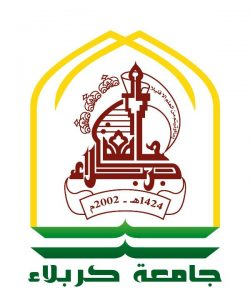## محمدحسين كاظم عباس

Abstract

This research presented isotropic hyper composite plates structures, which are made of (resin and fiber with powder as a reinforcement material). The basic item of this research is to improve the mechanical and dynamical properties of the composite structure. This item has been verified by adding the powder as a reinforcement material.

Square and rectangular hyper composite plates (Aspect ratio =1 and 1.5) are made with different volume fractions for powder (two types of powder are used which are glass and date palm nuts), resin and short fiber. Six different boundary conditions are used to support these plates.

The mechanical and dynamical properties of hyper composite models are calculated with considering these different parameters. The mechanical properties (modulus of elasticity, and yield stress) are calculated through the experimental (tensile test) and analytical considerations. A comparison between them is made with a percentage difference approximately 11%.

The dynamical properties of these different models are estimated numerically and experimentally. In addition, the analytical method is also used to estimate these dynamical properties for simply supported models only.

The composite plates are excited freely to calculate the value of the fundamental natural frequency. The simply supported models are analyzed numerically, analytically and are also tested experimentally; a comparison is made between them with approximate difference 14%. But, the other supports are analyzed numerically and experimentally (vibration test), and the results are compared. Also, these models are tested using a transient load in order to calculate the deflection and stresses analytically and numerically.

It is found that the mechanical properties of hyper composite plate are improved by increasing the volume fraction of powder or short fiber with decreasing the resin. Also, the natural frequency increases by increasing the volume fraction of powder or short fiber with decreasing the resin. However, it is found that deflections and stresses of the models were decreased by increasing the volume fraction of powder or short fiber with decreasing in the resin materials.

Finally, the comparison between glass powder and date palm nuts powder for same volume fraction of 30% short fiber, 10% powder, and 60% resin matrix composite plate showed that the yield stress of composite with date palm nuts powder is increased with about 30.81% than that of glass powder, but the modulus of elasticity is decreased with about 21.4%. Also, the comparison between glass powder and date palm nuts revealed that the values of fundamental natural frequency, deflection and stresses of the date palm nuts were higher than those for the composite plate containing glass powder.

Thus, the results encourage using this type of hyper composite plate safely in the engineering applications, where high loads are encountered in a wide range of operating frequencies.

Verification of the mechanical properties is made between the present experimental results and analytical results existing by , where the difference not exceeds 10%. The comparison of fundamental natural frequency for isotropic hyper composite plates between present experimental results and analytical results by  for simply supported plate where the maximum difference is about 11%.